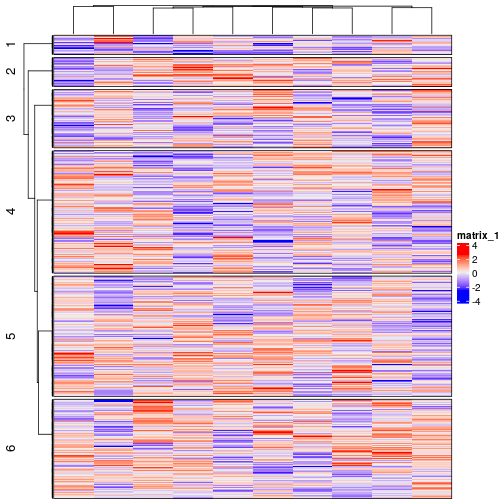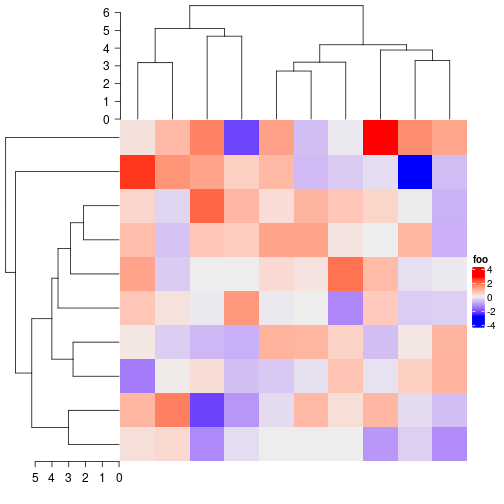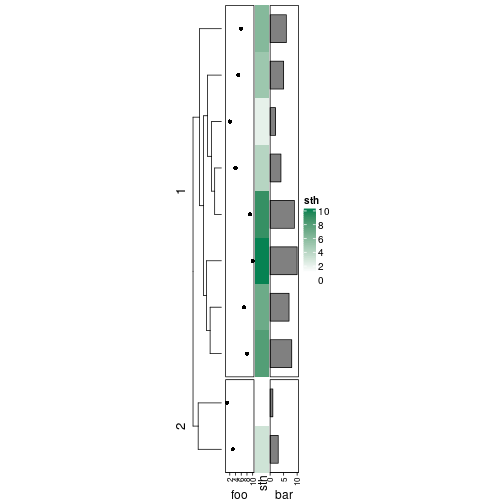### There is no plot coming out after running Heatmap() function.

In this case, you need to use `draw()` function explicitly. See https://jokergoo.github.io/ComplexHeatmap-reference/book/a-single-heatmap.html#plot-the-heatmap and https://jokergoo.github.io/ComplexHeatmap-reference/book/a-list-of-heatmaps.html#plot-the-heamtap-list.

### Retrieve orders and dendrograms.

For retrieving orders and dendrograms from a single heatmap. See https://jokergoo.github.io/ComplexHeatmap-reference/book/a-single-heatmap.html#get-orders-and-dendrograms-from-heatmap.

For retrieving orders and dendrograms from a list of heatmaps. See https://jokergoo.github.io/ComplexHeatmap-reference/book/a-list-of-heatmaps.html#get-orders-and-dendrograms-from-a-list-of-heatmaps.

### How should I control the height or width of the heatmap annotations?

For complex annotations generated by `anno_*()` functions, width or height should be set inside the `anno_*()` function, such as `anno_points(..., height = ...)`. The size of simple annotations is controlled by `anno_simple_size`. The `width`/`height` and `annotation_width`/`annotation_height` are used to adjust the size for multiple annotations which are put in one `HeatmapAnnotation` object. See https://jokergoo.github.io/ComplexHeatmap-reference/book/heatmap-annotations.html#multiple-annotations

### How should I control the axes of the annotations?

In the annotation functions `anno_*()`, the argument `axis_param` can be used to set the axes. The value should be a list and the default settings for axis can be get by:

``````default_axis_param("column")
default_axis_param("row")
``````

### How to control the style of legends?

The style of legends can be controlled by `heatmap_legend_param` in `Heatmap()`, or `annotation_legend_param` in `HeatmapAnnotation()`. The parameters for controlling legends are those arguments in `Legend()` function. See https://jokergoo.github.io/ComplexHeatmap-reference/book/legends.html#heatmap-and-annotation-legends.

### Some text are cut by the plotting region.

The layout of the ComplexHeatmap is not perfect that it is still possible some of the text are drawn out of the plotting region. In this case, you can set the `padding` argument in `draw()` function to increase the blank areas around the final plot. See https://jokergoo.github.io/ComplexHeatmap-reference/book/a-list-of-heatmaps.html#manually-increase-space-around-the-plot.

### Can the heatmaps be added vertically?

Yes, use `%v%` instead of `+`. See https://jokergoo.github.io/ComplexHeatmap-reference/book/a-list-of-heatmaps.html#vertical-concatenation.

### Does Heatmap title supports mathematical expression?

Yes, all the text-related elements (e.g. titles, row names, legend titles, legend labels, …) allow methematical expression.

### I have many heatmaps and I want to put them into different panels for a big figure for my paper.

You can set `newpage = FALSE` in `draw()` function and use `grid.layout()` to manage the layout of your panels.

``````pushViewport(viewport(layout = grid.layout(...)))
pushViewport(viewport(layout.pos.row = ..., layout.pos.col = ...))
draw(ht, newpage = FALSE) # or draw(ht_list, newpage = FALSE)
popViewport()
...
``````

But I more suggest to use `grid.grabExpr()` to directly capture the output of the heatmap and later draw the whole plot as a single graphic element by `grid.draw()`.

``````ht_grob = grid.grabExpr(draw(ht, ...))

pushViewport(viewport(layout = grid.layout(...)))
pushViewport(viewport(layout.pos.row = ..., layout.pos.col = ...))
grid.draw(ht_grob)
popViewport()
...
``````

### I have a matrix with too many rows and I want to simplify the row dendrogram.

You can first group your rows into several groups and make a group-level dendrogram on it. See following example:

``````m = matrix(rnorm(1000*10), nr = 1000)
hc = hclust(dist(m))
group = cutree(hc, k = 6)
Heatmap(m, cluster_rows = cluster_within_group(t(m), group),
row_split = 6, border = TRUE) # it would be better if also set row_split
``````### I have a matrix with huge nunmber of rows or columns, what is the efficient way to visualize it?

Heatmap is used to visualize the global patterns of your matrix while not every single row or column. I suggest to random sample rows or columns into a reasonable small number, and the final heatmap should look the same as if you still insist to use the full matrix.

### How to add axes for dendrograms?

You need to use `decorate_row_dend()` or `decorate_column_dend()` to manually add the axes. See following examples:

``````m = matrix(rnorm(100), 10)

ht = Heatmap(m, name = "foo",
row_dend_width = unit(4, "cm"),
column_dend_height = unit(4, "cm")
)
draw(ht, padding = unit(c(15, 2, 2, 2), "mm"))
decorate_column_dend("foo", {
grid.yaxis()
})
decorate_row_dend("foo", {
vp = current.viewport()
xscale = vp\$xscale
grid.xaxis(at = xscale - 0:5, label = 0:5)
})
``````Note for the left row dendrogram, the x-axis is from right to left, you need to self-define `at` and `label` in `grid.xaxis()` function.

You can also check `annotation_axis_grob()` function (later use `grid.draw()` to draw the axes) to draw a nicer axis.

### I set row_km/column_km and it gives me different k-means clusterings for different runs.

Yes, this is what it should be expected because k-means uses random start points and it might give different results for different runs. To solve this problem, you do either way as follows:

1. Always add `set.seed(...)` before making the heatmap. This makes sure the random seed is always the same for different runs.
2. Set `row_km_repeats`/`column_km_repeats` to run k-means multiple times to get a final consensus k-means clustering. Note you might still get different results, but the chance is much smaller than just running k-means once.

### I only want to draw dendrograms plus a list of annotations.

You need to assign the dendrograms to a zero-row/column matrix:

``````hc = hclust(dist(matrix(rnorm(100), 10)))
Heatmap(matrix(nc = 0, nr = 10), cluster_rows = hc,
right_annotation = rowAnnotation(
foo = anno_points(1:10),
sth = 1:10,
bar = anno_barplot(1:10)),
row_split = 2)
``````### I still have a problem with the package and I am lost in the ocean of the huge vignette.

The vignette (https://jokergoo.github.io/ComplexHeatmap-reference/book/) contains huge number of examples and plots showing different usage of the package. It is sometimes not easy to find the solution you are looking for. In this case, don't hesitate to write me an email. I am glad to answer all of your questions!

### Can I also add heatmaps produced by pheatmap()?

Yes, you can refer to https://jokergoo.github.io/2020/05/06/translate-from-pheatmap-to-complexheatmap/.